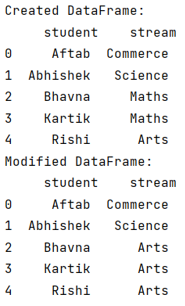# Replace whole string if it contains substring in pandas

Given a pandas dataframe, we have to replace whole string if it contains substring in it.
Submitted by Pranit Sharma, on September 14, 2022

Pandas is a special tool that allows us to perform complex manipulations of data effectively and efficiently. Inside pandas, we mostly deal with a dataset in the form of DataFrame. DataFrames are 2-dimensional data structures in pandas. DataFrames consist of rows, columns, and data.

A substring is a sequence of characters within a string that is contiguous. For example, "llo Wor" is a substring of "Hello World". The important point is sequence is different from sub-sequence, "loWor" is a subsequence of "Hello World", but not a substring.

## Problem statement

Suppose, we have a DataFrame that contains a string-type column and we need to filter the column based on a substring, if the value contains that particular substring, we need to replace the whole string.

## Pandas - Replacing whole string if it contains substring

For this purpose, we will use the str.contains() method to mask the rows that contain the old substring and then overwrite with the new value (i.e., new string to be replaced with old one). Consider the below code statement to achieve this task,

```df.loc[df['stream'].str.contains('aths'), 'stream'] = 'Arts'
```

Let us understand with the help of an example,

## Python program to replace whole string if it contains substring in pandas

```# Importing pandas package
import pandas as pd

# Creating a dictionary with equal elements
d = {
'student':['Aftab','Abhishek','Bhavna','Kartik','Rishi'],
'stream':['Commerce','Science','Maths','Maths','Arts']
}

# Creating a DataFrame
df = pd.DataFrame(d)

# Display DataFrame
print("Created DataFrame:\n",df)

# Filtering and replacing Stream column
df.loc[df['stream'].str.contains('aths'), 'stream'] = 'Arts'

# Display modified DataFrame
print("Modified DataFrame:\n",df)
```

## Output

The output of the above program is: